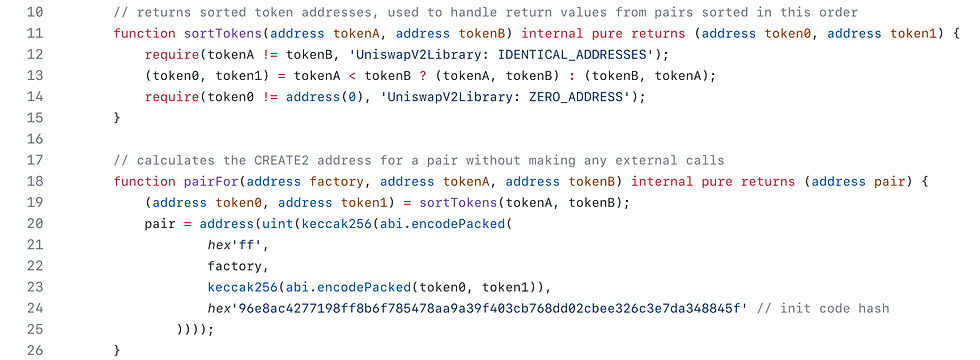top of page
• Jeffrey Scholz

# UniswapV2Library Code Walkthrough

## UniswapV2Library

The Uniswap V2 Library simplifies some interactions with pair contracts and is used heavily by the Router contracts. It contains eight functions that are not state-changing,. They are also handy for integrating Uniswap V2 from a smart contract.

## getAmountOut() and getAmountIn()

If we want to predict the amount of token y we will get if we supply a fixed amount of token x, then we can derive the amount out using the sequence below (ignoring fees for simplicity). Let x be the incoming token, y be the outgoing token, Δx be the amount coming in and Δy be the amount going outWith that in mind, the function for getAmountOut() in the UniswapV2Library.sol should be self-explanatory. Note that the numbers are scaled by 1,000 to account for the 0.3% fee. The derivation for getAmountIn() with fees is an exercise for the reader.## Chaining getAmountOut() to getAmountsOut() for pair hops

### getAmountsOut() and getAmountsIn()

If a trader supplies a sequence of pairs, (A, B), (B, C), (C, D) and iteratively calls getAmountOut starting with a certain amount of A, then the amount of token D that will be received can be predicted

The address of the UniswapV2 pair contract for each (A, B), (B, C), etc. is deterministically derived from the addresses of the tokens and the address of the factory that deployed the pair using the create2 function. Given two tokens (A, B) and a factory address, pairFor() derives the address of the pair UniswapV2 pool contract for that pair, using sortTokens() as a helper function.Now that we know the addresses for all the pairs, we can get the reserves of each one and predict how much tokens we will receive at the end of the chain of swaps. Below is the code for getAmountsOut (emphasis on “Amounts” instead of “Amount”). The function getAmountsIn simply does the same thing in reverse so we won’t show it here.

Note a couple things:

• The smart contract doesn’t figure out the optimal sequence of pairs on its own, it needs to be told the list of pairs to calculate the chain of swaps over. This is best done off-chain.

• It doesn’t just return the final token amountOut in the chain, but the amount out at every step.## getReserves()

The function getReserves is simply a wrapper around the function getReserves from the Uniswap V2 pair contract except that it also removes the timestamp when the price was last updated. The function for getReserves from the pair contract is also shown for easy comparison (purple comments).Core function## quote()

Recall that the price of an asset follows the following formula:This function returns the price of foo denominated in bar as of the last update. This function should be used with care as it is vulnerable to flash loan attacks.## Using UniswapV2Library

If you want to predict how much to put into or expect out of a trade, or a sequence of trades across pairs, the UniswapV2Library is the tool to use.

## Practice

Try out DamnVulnerableDefi Puppet V2. It should be easy for you to identify the security issue at this point.